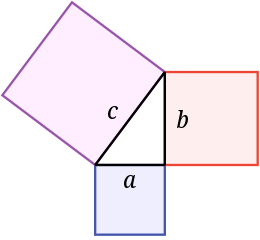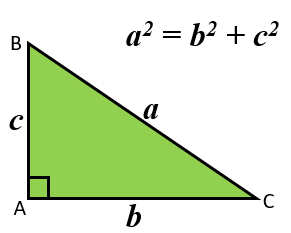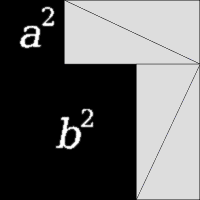## PythagorasPythagoras’ Theorem is one of the most important theorems in geometry.It states that the area of the square whose side is the hypotenuse (the side opposite the right angle) is equal to the sum of the areas of the squares on the other two sides.

Here are two proofs of Pythagoras’ Theorem: